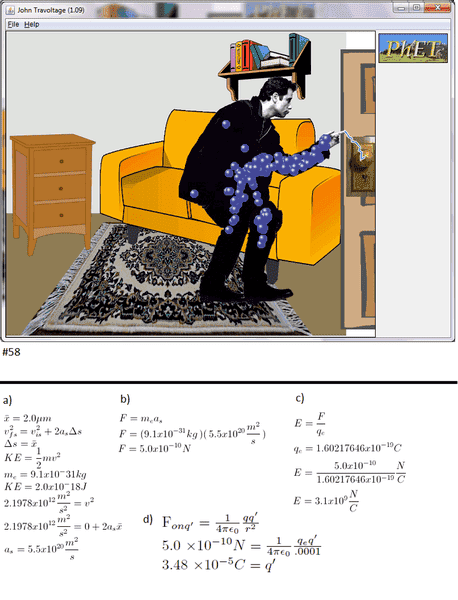# Electric field necessary to create a spark.

joe_cool2

## Homework Statement

a) The average distance an electron travels between collisions is 2.0$\mu$m. What acceleration must an electron have to gain 2.0 x 10-18 J of kinectic energy in this distance?

b) What force must act on an electron to give it the acceleration found in part a?

c) What strength electric field will exert this much force on an electron? This is the breakdown field strength?

d) Suppose a free electron in air is 1.0 cm away from a point charge. What minimum charge qmin must this point charge have to cause a breakdown of the air and create a spark?

## Homework Equations

$$F_{on q'} = \frac{1}{4\pi\epsilon_{0}}\frac{qq'}{r^2}\\ v^2 = v_0^2 + 2 a \Delta x\\ K = \frac{1}{2}m \ v^2$$

## The Attempt at a Solution

I did this problem on the computer, summing it all up in a .png with some images rendered by my LaTeX editor that represent the work. The picture is just to prove to my prof that I did this certain activity. Did I do anything wrong here?Last edited:

Staff Emeritus
Homework Helper
Gold Member

## Homework Statement

a) The average distance an electron travels between collisions is 2.0$\mu$m. What acceleration must an electron have to gain 2.0 x 10-18 J of kinectic energy in this distance?

b) What force must act on an electron to give it the acceleration found in part a?

c) What strength electric field will exert this much force on an electron? This is the breakdown field strength?

d) Suppose a free electron in air is 1.0 cm away from a point charge. What minimum charge qmin must this point charge have to cause a breakdown of the air and create a spark?

## Homework Equations

$$F_{on q'} = \frac{1}{4\pi\epsilon_{0}}\frac{qq'}{r^2}\\ v^2 = v_0^2 + 2 a \Delta x\\ K = \frac{1}{2}m \ v^2$$

## The Attempt at a Solution

I did this problem on the computer, summing it all up in a .png with some images rendered by my LaTeX editor that represent the work. The picture is just to prove to my prof that I did this certain activity. Did I do anything wrong here?

[ IMG]http://s15.postimage.org/kkqh30ixn/physics26_58.png[/PLAIN]
You can do part b, simply by invoking the work-energy theorem.

The force you found is hundreds of times too large.